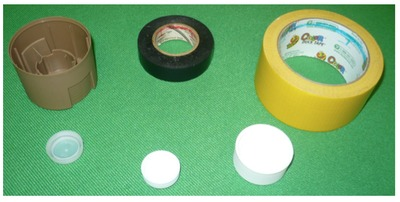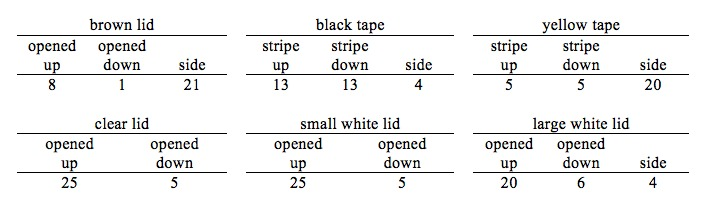# Tossing Cylinders

Alignments to Content Standards: 7.SP.C.6

Think about a cylindrical (or cylinder-like) object, such as a bottle lid or a roll of tape. Some possible objects are shown in the picture below. Suppose you were to toss one of these objects into the air and observe its landing position once it reaches the floor.1. For your object, what are the possible outcomes of this experiment?
2. Make a guess – what are the probabilities of each of the possible outcomes?
3. Toss the object into the air and record the outcome. Repeat this process 25 to 30 times.
4. Determine the experimental probability of each outcome. How does this experimental probability compare to your guess from part b? Based on this information, would you like to change your guess?
5. Repeat this activity with a different cylindrical (or cylinder-like) object that you think has a greater probability of landing on its side than your first object.

## IM Commentary

The purpose of this task is to provide students with the opportunity to determine experimental probabilities by collecting data. The cylindrical objects used in this task typically have three different resting positions, but not all of these may be equally likely, and some may be extremely unlikely or impossible when the object is tossed. Furthermore, obtaining the probabilities of the outcomes is perhaps only possible through the use of long-run relative frequencies. This is because these cylinders do not have the same types of symmetries as objects that are often used as dice, such as cubes or tetrahedrons, where each outcome is equally likely.

1. The teacher may use a variety of different objects, or several identical objects.
2. I colored the inside of the roll of tape closer to one side to differentiate “stripe up” from a “stripe down.” Both tape rolls are shown in “stripe up” position.
3. If the object strikes something (such as a book, desk, or student) before coming to rest, do not count that toss.

For more information on this topic, please see the following article: Jones, D. L. (2009, February). Examining cylindrical dice. Mathematics Teacher, 102(6), 420-424.

## Solution

1. For the large white and brown lids shown in the picture, the three possible outcomes are “opened up,” “opened down,” and “side.”

The two smallest lids would not balance on their sides, so the two possible outcomes are “opened up” and “opened down.”

The rolls of tape had three possible outcomes, which I called “stripe up,” “stripe down,” and “side.”

2. Guesses will likely vary. Students may estimate a greater probability for “opened up” than “opened down” because of the difference in weight in a lid. For rolls of tape, estimates for “top” and “bottom” may be the same because of ideas of symmetry.
3. Below is a table with data from 30 tosses of each of the cylinders shown above.4. The experimental probabilities based on the data in part c are calculated by dividing the frequency of each outcome by the total number of tosses (in this case, 30).

These probabilities may be very different, or very similar to, the guesses made in part b. The key point in this activity is that the estimates should be informed by the actual data.

5. Students will likely look for an object where it is possible for the object to land on its side, and has a greater height-to-diameter ratio than the first object.